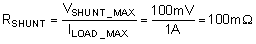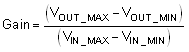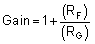SBOS878D July   2017  – October 2019

PRODUCTION DATA.

1. Features
2. Applications
3. Description
1.     Device Images
4. Revision History
5. Device Comparison Table
6. Pin Configuration and Functions
7. Specifications
8. Detailed Description
1. 8.1 Overview
2. 8.2 Functional Block Diagram
3. 8.3 Feature Description
4. 8.4 Device Functional Modes
9. Application and Implementation
1. 9.1 Application Information
2. 9.2 Typical Application
10. 10Power Supply Recommendations
11. 11Layout
12. 12Device and Documentation Support
13. 13Mechanical, Packaging, and Orderable Information

#### Package Options

Refer to the PDF data sheet for device specific package drawings

• DBV|5
• DCK|5
• DCK|5

#### 9.2.2 Detailed Design Procedure

The transfer function of the circuit in Figure 35 is shown in Equation 1.

Equation 1.The load current (ILOAD) produces a voltage drop across the shunt resistor (RSHUNT). The load current is set from 0 A to 1 A. To keep the shunt voltage below 100 mV at maximum load current, the largest shunt resistor is defined using Equation 2.

Equation 2.Using Equation 2, RSHUNT is 100 mΩ. The voltage drop produced by ILOAD and RSHUNT is amplified by the TSV91x to produce an output voltage of approximately 0 V to 4.95 V. The gain required by the TSV91x to produce the necessary output voltage is calculated using Equation 3:

Equation 3.Using Equation 3, the required gain is calculated to be 49.5 V/V, which is set with resistors RF and RG. Equation 4 is used to size the resistors, RF and RG, to set the gain of the TSV91x to 49.5 V/V.

Equation 4.Selecting RF as 165 kΩ and RG as 3.4 kΩ provides a combination that equals roughly 49.5 V/V. Figure 36 shows the measured transfer function of the circuit shown in Figure 35.